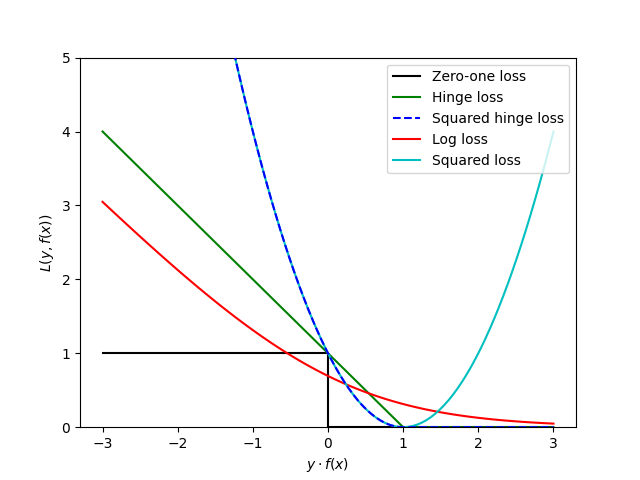# SGD: Convex Loss Functions¶

print(__doc__)

import numpy as np
import pylab as pl
from lightning.impl.sgd import Hinge
from lightning.impl.sgd import SquaredHinge
from lightning.impl.sgd import Log
from lightning.impl.sgd import SquaredLoss


Define loss funcitons

xmin, xmax = -3, 3
hinge = Hinge(1)
squared_hinge = SquaredHinge()
log = Log()
squared_loss = SquaredLoss()


Plot loss funcitons

xx = np.linspace(xmin, xmax, 100)
pl.plot([xmin, 0, 0, xmax], [1, 1, 0, 0], 'k-',
label="Zero-one loss")
pl.plot(xx, [hinge.loss(x, 1) for x in xx], 'g-',
label="Hinge loss")
pl.plot(xx, [squared_hinge.loss(x, 1) for x in xx], 'b--',
label="Squared hinge loss", zorder=3)
pl.plot(xx, [log.loss(x, 1) for x in xx], 'r-',
label="Log loss")
pl.plot(xx, [2.0*squared_loss.loss(x, 1) for x in xx], 'c-',
label="Squared loss")
pl.ylim((0, 5))
pl.legend(loc="upper right")
pl.xlabel(r"$y \cdot f(x)$")
pl.ylabel("$L(y, f(x))$")
pl.show()Total running time of the script: ( 0 minutes 0.367 seconds)

Gallery generated by Sphinx-Gallery